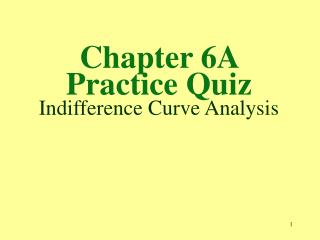DownloadDownload PresentationChapter 6A Practice Quiz Indifference Curve Analysis

# Chapter 6A Practice Quiz Indifference Curve Analysis

Télécharger la présentation## Chapter 6A Practice Quiz Indifference Curve Analysis

- - - - - - - - - - - - - - - - - - - - - - - - - - - E N D - - - - - - - - - - - - - - - - - - - - - - - - - - -
##### Presentation Transcript

1. Chapter 6A Practice Quiz Indifference Curve Analysis

2. 1. An indifference curve consists of quantity combination of two goods that yield a.equal marginal utilities. b. negative marginal utilities. c. the same price ratios. d. the same total satisfaction. D. Answers “equal marginal utilities” and “negative marginal utilities” involve a change in total utility (marginal utility), and answer “the same price ratios”does not define an indifference curve.

3. 2. The absolute value of the slope of an indifference curve is called the a. marginal rate of transformation. b. transitivity slope. c. indifference rate of preference. d. marginal rate of substitution. D. The other answers are nonsense terms.

4. 3. The slope of the indifference curve for goods X and Y is called the marginal a. product rate. b. rate of transformation. c. rate of substitution. d. rate of utility. C. The other answers are nonsense terms.

5. 4. Given the prices of two goods, all quantity combinations inside the budget line are a. indifferent. b. efficient. c. unattainable. d. attainable. D. See Exhibit A-3 in the text.

6. 5. Assume the price of good X is PX, price of good Y is PY, and B is the budget. . The formula for the budget line for these two goods is a. PYQY/PXQX. b. PXB + PYB = B. c. PXX + PYY = B. d. (1 – PY/B)PX. C. See Exhibit A-3 in the text.

7. 6. The ratio of the price of good X on the horizontal axis to the price of good Y on the vertical axis is the ______ of the budget line. a. marginal rate b. slope c. marginal utility d. equalization rate B. Computing the slope of the budget line results in the ratio of the price of the good on the horizontal axis divided by the price of the good on the vertical axis.

8. 7. Assume Px is the price of good X on the horizontal axis and Py is the price of good Y on the vertical axis. The slope of the budget line equals a. Py/PxY. b. PyQy/PxQx. c. (1 - Py/Px). d. Px/Py. D. Computing the slope of the budget line results in the ratio of the price of the good on the horizontal axis divided by the price of the good on the vertical axis.

9. 8. Consumer equilibrium occurs where the budget line is tangent to the a. lowest possible indifference curve. b. highest possible indifference curve. c. utility-maximizing indifference curve. d. utility-equalization indifference curve. B. High indifference curves yield higher satisfaction, but they are unattainable beyond the tangency to a given budget line.

10. 9. Only at the point of consumer equilibrium does the marginal rate of substitution (MRS) equal the a. slope of the budget line. b. slope of the indifference curve. c. price ratio. d. all of the above. D. All of the answers are correct.

11. 10. At point A in Exhibit A-6, consumers would be a. spending all of their income but not maximizing total utility. b. spending all of their income and maximizing total utility. c. maximizing total utility without spending all of their income. d. none of the above. A. Point A is a point on the budget line so, given the prices of good x and y, the entire amount of income is spent. However, at point C the consumer would be on higher indifference curve I2.

12. 11. The consumer equilibrium shown in Exhibit A-6 is located at point a. A. b. B. c. C. d. D. C. Consumer equilibrium occurs at point C where the budget line is tangent to the highest attainable indifference curve (I2).

13. 12. In Exhibit A-6, point D is a. a consumer equilibrium. b. unattainable given the consumer’s current budget constraint. c. a point that does not exhaust all of the consumer’s income. d. none of the above. B. At point D, the budget line constraint does not intersect point D on indifference curve I3.

14. 13. Assume that a consumer’s preference is for two goods X and Y in Exhibit A-6. By holding the price of Y and money income constant while varying the price of X, it is possible to derivea. The demand curve for X. b. The demand curve for Y. c. The demand curve for both X and Y. d. None of the above. A. At a price for good X of \$6, the quantity demanded is obtained at point C on the highest possible indifference curve I2. At a price for good X of \$5, the quantity demanded is obtained at point D on the new highest possible indifference curve I3 created by the outward shift in the budget line.